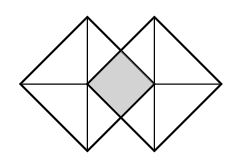### Home > CCA2 > Chapter Ch3 > Lesson 3.2.5 > Problem3-125

3-125.

Two congruent overlapping squares are shown at right. If a point inside the figure is chosen at random, what is the probability that it will not be in the shaded region? Homework Help ✎

Draw the figure on your graph paper to scale. Divide the shape into equal parts. Count how many are not shaded.

$\frac {6}{7}$